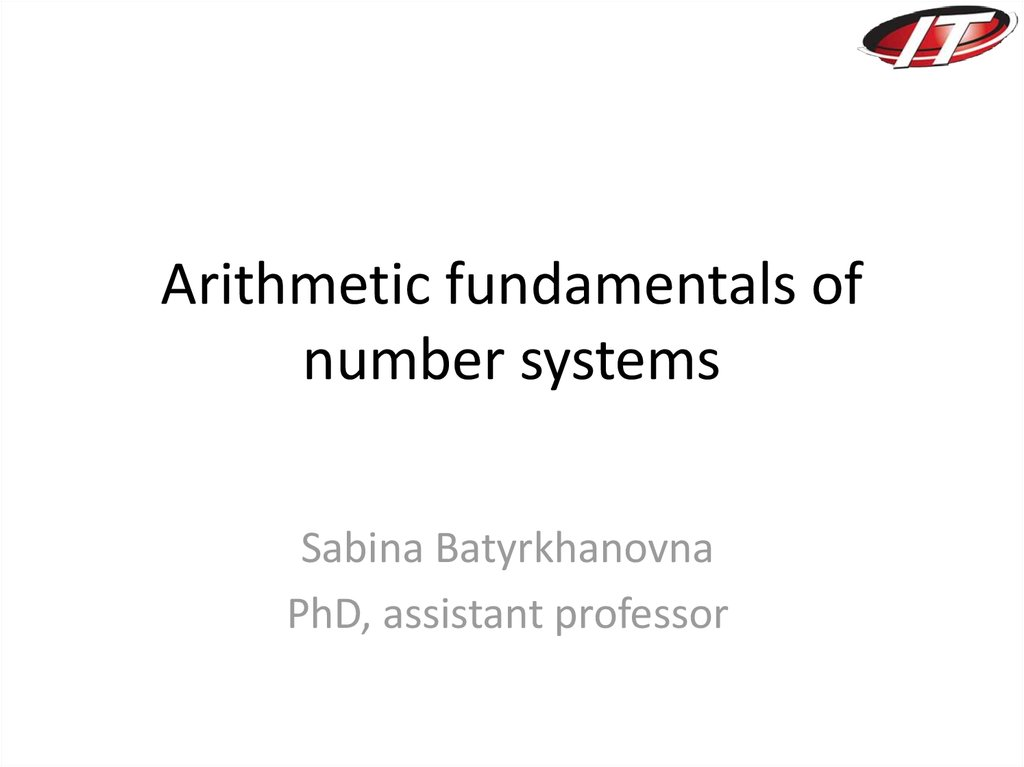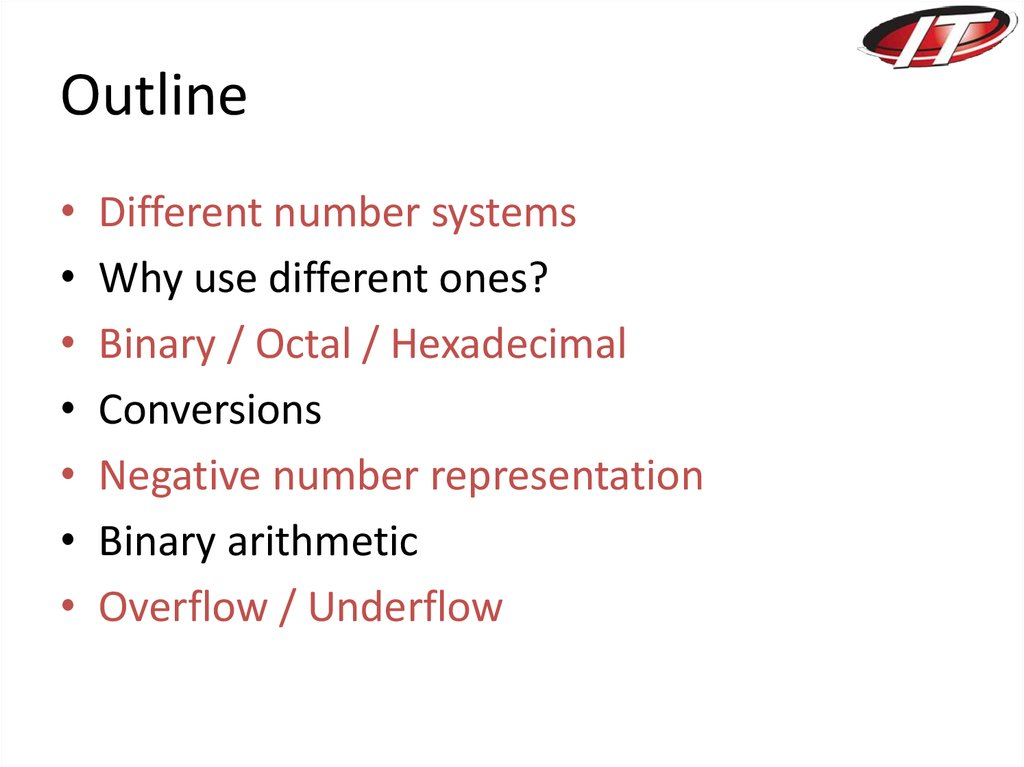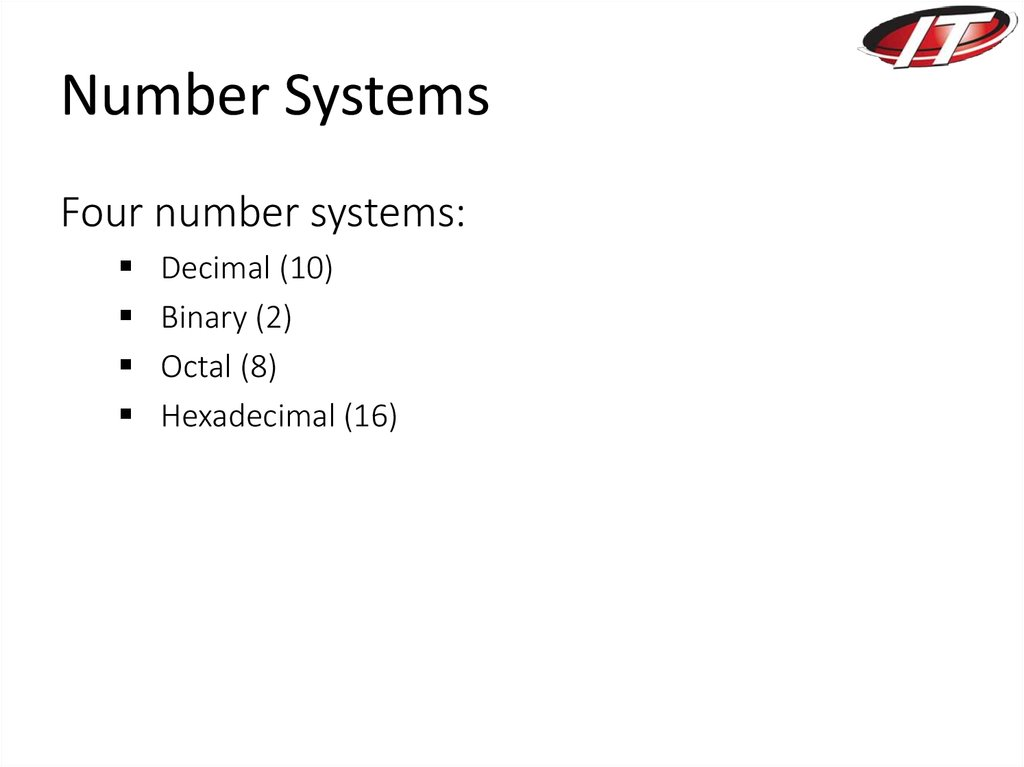# Arithmetic fundamentals of number systems

## 1. Arithmetic fundamentals of number systems

Sabina Batyrkhanovna
PhD, assistant professor

## 2. Outline

Different number systems
Why use different ones?
Conversions
Negative number representation
Binary arithmetic
Overflow / Underflow

## 3. Number Systems

Four number systems:
Decimal (10)
Binary (2)
Octal (8)

## 4. Binary numbers

• Computers work only on two states
– On
– Off
• Basic memory elements hold only two states
– Zero / One
• Thus a number system with two elements {0,1}
• A binary digit – bit !

## 5. Decimal numbers

1439 = 1 x 103 + 4 x 102 + 3 x 101 + 9 x 100
Thousands
Hundreds
Tens
Ones

## 6. Binary Decimal

1101 = 1 x 23 + 1 x 22 + 0 x 21 + 1 x 20
=1x8+1x4+0x2+1x1
=8+4+0+1
(1101)2 = (13)10
1, 2, 4, 8, 16, 32, 64, 128, 256, 512, …

2
2
13
6
2
2
3
1
0
(13)10 = (1101)2
LSB
1
0
1
1
MSB

## 8. Octal Decimal

137 = 1 x 82 + 3 x 81 + 7 x 80
= 1 x 64 + 3 x 8 + 7 x 1
= 64 + 24 + 7
(137)8 = (95)10
• Digits used in Octal number system – 0 to 7

8
8
95
11
7
3
8
1
0
1
(95)10 = (137)8
LSP
MSP

## 10. Hex Decimal

BAD = 11 x 162 + 10 x 161 + 13 x 160
= 11 x 256 + 10 x 16 + 13 x 1
= 2816 + 160 + 13
A = 10, B = 11, C = 12, D = 13, E = 14, F = 15

16
16
2989
186
16
11
0
13
10
11
LSP
MSP

## 12. Why octal or hex?

• Ease of use and conversion
• Three bits make one octal digit
111 010 110 101
7 2 6 5 => 7265 in octal
• Four bits make one hexadecimal digit
4 bits = nibble
1110 1011 0101
E B
5
=> EB5 in hex

## 14. A Brief History of Roman Numerals

• Roman numerals originated in ancient Rome. This
ancient counting system is believed to have started
with the ancient Etruscans.
• The symbol for one in the roman numeral system
probably represented a single tally mark which people
would notch into wood or dirt to keep track of items
or events they were counting. It would also be easy to
write on a wax tablet.

## 15. Arabic numbers → Roman numerals conversion

• Roman numerals are written as combinations
of seven letters.
I=1 V=5
X = 10
L = 50
C = 100
D =500
M = 1000
• The letters can be written as capital (XVI) or
lower-case letters (xvi).

## 16. As a general guide

subtracting numbers like this:• 11=10+1 = XI
9 = 10 – 1 = IX
• 40 = 50 –10 = XL
• If you want to say 1,100 in Roman Numerals,
you would say M for 1000 and then put a C
after it for 100; 1,100 = MC
• 900 =1000 – 100 so the C comes before M =
CM

## 17. Some more examples:

VIII = 5+3 = 8
XIX = 10+ 9 = 19
(Remember 9 is always = IX (1 less than 10)
XL = 50-10 = 40
XC = 100-10 = 90
Try these on whiteboards:
7 = 12 = 15 = 20 =

7 = VII
12 = XII
15 = XV
20 = XX

• 17 = 22 =
• 32 =
35 =
• 44 = 49 =
26 = 29 = 30 =
50 = 40 =
58 =
60 =

## 20. Were you correct?

• 17=XVII 22=XXII 26=XXVI 29=XXIX
• 30=XXX 32=XXXII
• 50=L
35=XXXV
40=XL
• 44=XLIV 49=XLIX 58=LVIII 60=LX

## 21. Some more large numbers to try:

• 600 =
700 =
• 1000 =
900 =
• 1600 =
• 2000 =
1700 =
800 =
1900 =

• 600 = DC 700 = DCC
800 = DCCC
• 1000 = M 900 = CM
• 1600 =MDC
1700 =MDCC
• 1900 = MCM
2000 =MM

## 23. The last one

• Can you convert 2017?
• MMXVII
Now try to write today’s date.
Day / Month / Year
• Well done. You are a Roman Numeral
Converter!

## 24. Binary Arithmetic

• Subtraction

B
A
+
0
1
0101 (5)
0
0
1
+ 1001 (9)
1
1
10
1110 (14)
The carry out of the MSB is neglected

## 26. Subtraction

Like normal decimal subtraction
B
A
-
0
1
1001 (9)
0
0
11
- 0101 (5)
1
1
0
0100 (4)
A borrow (shown in red) from the MSB implies a negative